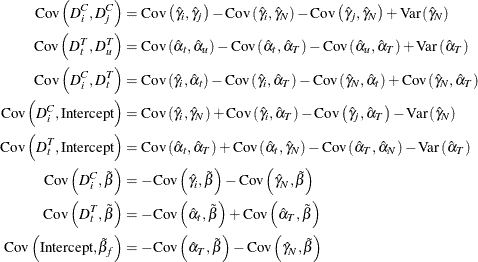The PANEL Procedure

Unbalanced Panels

Subsections:

Let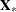and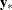be the independent and dependent variables arranged by time and by cross section within each time period. (Note that the input data set used by the PANEL procedure must be sorted by cross section and then by time within each cross section.) Let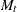be the number of cross sections observed in yearand let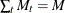. Let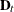be the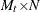matrix obtained from the
identity matrix from which rows that correspond to cross sections not observed at timehave been omitted. Consider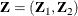where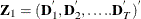and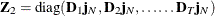. The matrix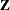gives the dummy variable structure for the two-way model.

Let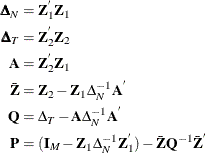The estimate of the regression slope coefficients is given by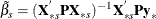where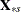is thematrix without the vector of 1s.

The estimator of the error variance is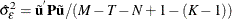where the residuals are given by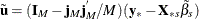if there is an intercept in the model and by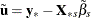if there is no intercept.

The actual implementation is quite different from the theory. The PANEL procedure transforms all series using the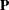matrix.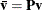The variable being transformed is, which could beor any column of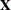. After the data are properly transformed, OLS is run on the resulting series.

Given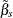, the next step is estimating the cross-sectional and time effects. Given that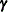is the column vector of cross-sectional effects and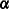is the column vector of time effects,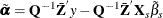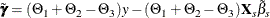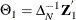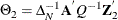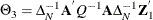Given the cross-sectional and time effects, the next step is to derive the associated dummy variables. Using the NOINT option, the following equations give the dummy variables: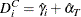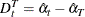When an intercept is desired, the equations for dummy variables and intercept are: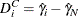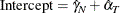The calculation of the covariance matrix is as follows: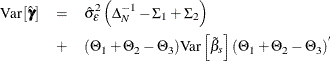where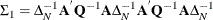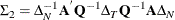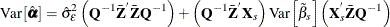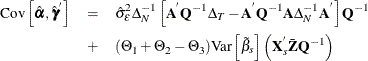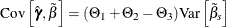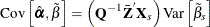Now you work out the variance covariance estimates for the dummy variables.

Variance Covariance of Dummy Variables with No Intercept

The variances and covariances of the dummy variables are given under the NOINT selection as follows: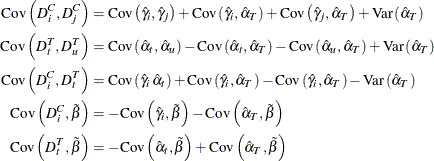Variance Covariance of Dummy Variables with Intercept

The variances and covariances of the dummy variables are given as follows when the intercept is included: